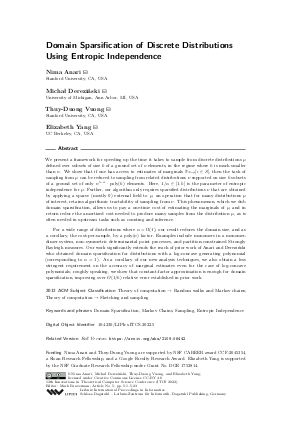Document# Domain Sparsification of Discrete Distributions Using Entropic Independence

### Authors Nima Anari, Michał Dereziński, Thuy-Duong Vuong, Elizabeth Yang## File

LIPIcs.ITCS.2022.5.pdf
• Filesize: 0.74 MB
• 23 pages

## Cite As

Nima Anari, Michał Dereziński, Thuy-Duong Vuong, and Elizabeth Yang. Domain Sparsification of Discrete Distributions Using Entropic Independence. In 13th Innovations in Theoretical Computer Science Conference (ITCS 2022). Leibniz International Proceedings in Informatics (LIPIcs), Volume 215, pp. 5:1-5:23, Schloss Dagstuhl - Leibniz-Zentrum für Informatik (2022)
https://doi.org/10.4230/LIPIcs.ITCS.2022.5

## Abstract

We present a framework for speeding up the time it takes to sample from discrete distributions μ defined over subsets of size k of a ground set of n elements, in the regime where k is much smaller than n. We show that if one has access to estimates of marginals P_{S∼ μ} {i ∈ S}, then the task of sampling from μ can be reduced to sampling from related distributions ν supported on size k subsets of a ground set of only n^{1-α}⋅ poly(k) elements. Here, 1/α ∈ [1, k] is the parameter of entropic independence for μ. Further, our algorithm only requires sparsified distributions ν that are obtained by applying a sparse (mostly 0) external field to μ, an operation that for many distributions μ of interest, retains algorithmic tractability of sampling from ν. This phenomenon, which we dub domain sparsification, allows us to pay a one-time cost of estimating the marginals of μ, and in return reduce the amortized cost needed to produce many samples from the distribution μ, as is often needed in upstream tasks such as counting and inference. For a wide range of distributions where α = Ω(1), our result reduces the domain size, and as a corollary, the cost-per-sample, by a poly(n) factor. Examples include monomers in a monomer-dimer system, non-symmetric determinantal point processes, and partition-constrained Strongly Rayleigh measures. Our work significantly extends the reach of prior work of Anari and Dereziński who obtained domain sparsification for distributions with a log-concave generating polynomial (corresponding to α = 1). As a corollary of our new analysis techniques, we also obtain a less stringent requirement on the accuracy of marginal estimates even for the case of log-concave polynomials; roughly speaking, we show that constant-factor approximation is enough for domain sparsification, improving over O(1/k) relative error established in prior work.

## Subject Classification

##### ACM Subject Classification
• Theory of computation → Random walks and Markov chains
• Theory of computation → Sketching and sampling
##### Keywords
• Domain Sparsification
• Markov Chains
• Sampling
• Entropic Independence

## Metrics

• Access Statistics
• Total Accesses (updated on a weekly basis)
0